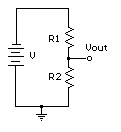## Voltage Divider CalculatorEnter a set of known values sufficient to define the circuit and then calculate the unknown values. Inputs and outputs are good only when 4 decimal places or less. Answers below four decimal places round to zero. Inputs and outputs are either Ohms Amps and Watts or kOhms, mAmps, and mWatts

 BatteryVoltage Current(A/mA) R1(Ohm/kOhm) R2(Ohm/kOhm) VR2 out(Volts) R1 W/mW = = W/mW R2

Return